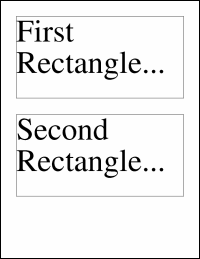Type Default Read Only Description [C#] `Rectangle` [Visual Basic] `Rectangle` n/a No The System.Drawing.Rectangle.Notes

 The rectangle as a System.Drawing Rectangle. Windows coordinates are measured in distances from the top left of the drawing surface while PDF coordinates are measured from the bottom left. So when you use this property the coordinates must be re-mapped. This needs to be done in the context of a containing object. Properties such as the Doc.Rect and the XImage.Selection have containers but others such as the Doc.MediaBox do not. In these cases the rectangles are assumed to contain themselves. You may find it easier to work with .NET Rectangles than PDF rectangles. However remember that operations such as Transforms work on the underlying PDF coordinates and not on the abstracted Windows coordinates.Example

 The following code adds two blocks of text to a document. The positioning is done using standard .NET Rectangles. [C#] ```Doc theDoc = new Doc(); theDoc.FontSize = 96; Rectangle rc = theDoc.MediaBox.Rectangle; rc.Inflate(-50, -50); rc.Height = 250; theDoc.Rect.Rectangle = rc; theDoc.FrameRect(); theDoc.AddText("First Rectangle..."); rc.Offset(0, 300); theDoc.Rect.Rectangle = rc; theDoc.FrameRect(); theDoc.AddText("Second Rectangle..."); theDoc.Save(Server.MapPath("xrectrectangle.pdf")); theDoc.Clear();``` [Visual Basic] ```Dim theDoc As Doc = New Doc() theDoc.FontSize = 96 Dim rc As Rectangle = theDoc.MediaBox.Rectangle rc.Inflate(-50, -50) rc.Height = 250 theDoc.Rect.Rectangle = rc theDoc.FrameRect() theDoc.AddText("First Rectangle...") rc.Offset(0, 300) theDoc.Rect.Rectangle = rc theDoc.FrameRect() theDoc.AddText("Second Rectangle...") theDoc.Save(Server.MapPath("xrectrectangle.pdf")) theDoc.Clear()```xrectrectangle.pdf